´

The q-analog of the Kramer-Mesner matrix

The next step in the construction is the evaluation of the entries of the Kramer-Mesner matrices. We achieve this goal by using the information obtained during the ladder game.

We can restrict attention to Kramer-Mesner matrices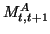, because of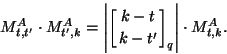(The analogous equation -- with binomial coefficients instead of the Gaussian numbers -- holds for designs on sets, the proof of it is easily generalized to the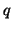-analog.

The matrixcan be obtained as follows: We assume the representatives of the double cosets of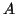and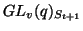, representatives of the double cosets ofand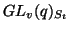and the corresponding orbit graph. Now the entry ofindexed by the orbits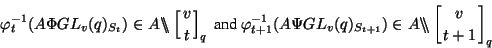is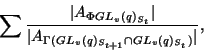where the sum is over all double cosets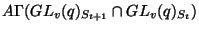which are connected with the double coset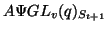and which are connected with the double coset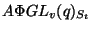at the same time.

If we take our last example then we get the Kramer-Mesner matrix: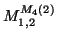(3,0,4) (3,1,2,) (3,2,6) (3,3,6) (3,4,8) (3,5,4) (5,0,6) 6/2 6/2 0 6/6 0 0 (5,1,4) 4/4 4/2 4/2 0 4/4 4/4 (5,2,6) 0 6/2 0 6/6 0 6/2 (5,3,24) 0 0 0 24/6 24/8 0

,

and thus the-analog of the Kramer-Mesner matrix turns out to be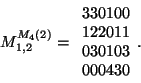Back to the title page

University of Bayreuth -